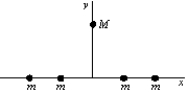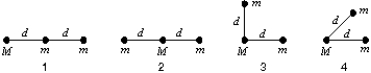# Fundamentals of Physics Study Set 2

Physics & Astronomy

## Quiz 13 : GravitationStudy FlashcardsLooking for Introductory Physics Homework Help?

## Quiz 13 :Gravitation

Question TypeLet M denote the mass of Earth and let R denote its radius.The ratio g/G at Earth's surface is:
Free
Multiple Choice

B

Tags
Choose question tagThe mass of a hypothetical planet is 1/100 that of Earth and its radius is 1/4 that of Earth.If a person weighs 600 N on Earth, what would he weigh on this planet?
Free
Multiple Choice

C

Tags
Choose question tagIn the formula F = Gm1m2/r2, the quantity G:
Free
Multiple Choice

D

Tags
Choose question tagAn astronaut finishes some work on the outside of his satellite, which is in circular orbit around the Earth.He leaves his wrench outside the satellite.If there is no air resistance, the wrench will:
Multiple Choice
Tags
Choose question tagThe mass of an object:
Multiple Choice
Tags
Choose question tagFour particles, each with mass m, are arranged symmetrically about the origin on the x axis.A fifth particle, with mass M, is on the y axis.The direction of the gravitational force on M is:Multiple Choice
Tags
Choose question tagAn artificial satellite of the Earth releases a bomb.Neglecting air resistance, the bomb will:
Multiple Choice
Tags
Choose question tagIf Earth were to rotate only 100 times per year about its axis:
Multiple Choice
Tags
Choose question tagEarth exerts a gravitational force on the Moon, keeping it in its orbit.The reaction to this force, in the sense of Newton's third law, is:
Multiple Choice
Tags
Choose question tagSuppose you have a pendulum clock which keeps correct time on Earth (acceleration due to gravity = 9.8 m/s2).Without changing the clock, you take it to the Moon (acceleration due to gravity = 1.6 m/s2).For every hour interval (on Earth)the Moon clock will record:
Multiple Choice
Tags
Choose question tagLet F1 be the magnitude of the gravitational force exerted on the Sun by Earth and F2 be the magnitude of the force exerted on Earth by the Sun.Then:
Multiple Choice
Tags
Choose question tagThe magnitude of the acceleration of a planet in orbit around the Sun is proportional to:
Multiple Choice
Tags
Choose question tagAn astronaut on the Moon simultaneously drops a feather and a hammer.The fact that they land together shows that:
Multiple Choice
Tags
Choose question tagThe gravitational constant G has the derived units
Multiple Choice
Tags
Choose question tagAn object at the surface of Earth (at a distance R from the center of Earth)weighs 90 N.Its weight at a distance 3R from the center of Earth is:
Multiple Choice
Tags
Choose question tagSuitable units for the gravitational constant G are:
Multiple Choice
Tags
Choose question tagMars has a mass of about 0.1075 times the mass of Earth and a diameter of about 0.533 times the diameter of Earth.The acceleration of a body falling near the surface of Mars is about:
Multiple Choice
Tags
Choose question tagAn object is raised from the surface of Earth to a height of two Earth radii above Earth.Then:
Multiple Choice
Tags
Choose question tagThe approximate value of g at an altitude above Earth equal to one Earth diameter is:
Multiple ChoiceThree particles, two with mass m and one mass M, might be arranged in any of the four configurations known below.Rank the configurations according to the magnitude of the gravitational force on M, least to greatest.